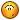# como reducir el drop de esta quest?

## Recommended Posts

quest special_drop1 begin
state start begin
when 20078.chat."Evento de pelotas de futbol" with pc.is_gm() begin
say_title("Empezar el evento")
say("Yushi2")
say("Quieres comenzar el evento?")
say("los monstruos que maten los users")
say("les dara unos items especiales")
say("")
say_reward("Que quieres hacer??")

local evento = select ("Actvarlo" , "Desactivarlo" ,  "Salir")
if evento == 1 then

game.set_event_flag("pelotafut_drop", 1)
notice_all("El evento de pelotas de futbol a comenzado en pandora solo los chicos podran abrir el cofre")
notice_all("Solo los personajes del 1 al 99 les caera el items")
elseif evento == 2 then

game.set_event_flag("pelotafut_drop", 0)
notice_all("El evento pelotas de futbol terminado en pandora")
elseif evento == 3 then
return
end
end
when kill with not npc.is_pc() and game.get_event_flag("pelotafut_drop") == 1 begin
local s = number(1, 200)
if s <= 2 then
if pc.get_level() >= 1 and pc.get_level() <= 99 then
game.drop_item(50096)
return
end
elseif s <= 2 then
if pc.get_level() >= 100 and pc.get_level() <= 101 then
game.drop_item(50096)
return
end

elseif s <= 2 then
if pc.get_level() >= 102 and pc.get_level() <= 103 then
game.drop_item(50096)
return
end

else if s <= 2 then
if pc.get_level() >= 104 and pc.get_level() <= 105 then
game.drop_item(50096)
return
end

elseif s <= 1 then
if pc.get_level() >= 105 and pc.get_level() <= 105 then
game.drop_item(50096)
end
end
end

end
end
end

tengo que reducir el numero llamado then¿ es el del drop o cual gracias _##### Share on other sites

quest special_drop1 begin
state start begin
when 20078.chat."Evento de pelotas de futbol" with pc.is_gm() begin
say_title("Empezar el evento")
say("Yushi2")
say("Quieres comenzar el evento?")
say("los monstruos que maten los users")
say("les dara unos items especiales")
say("")
say_reward("Que quieres hacer??")

local evento = select ("Actvarlo" , "Desactivarlo" ,  "Salir")
if evento == 1 then

game.set_event_flag("pelotafut_drop", 1)
notice_all("El evento de pelotas de futbol a comenzado en pandora solo los chicos podran abrir el cofre")
notice_all("Solo los personajes del 1 al 99 les caera el items")
elseif evento == 2 then

game.set_event_flag("pelotafut_drop", 0)
notice_all("El evento pelotas de futbol terminado en pandora")
elseif evento == 3 then
return
end
end
when kill with not npc.is_pc() and game.get_event_flag("pelotafut_drop") == 1 begin
local s = number(1, 200)
if s <= 2 then
if pc.get_level() >= 1 and pc.get_level() <= 99 then
game.drop_item(50096)
return
end
elseif s <= 2 then
if pc.get_level() >= 100 and pc.get_level() <= 101 then
game.drop_item(50096)
return
end

elseif s <= 2 then
if pc.get_level() >= 102 and pc.get_level() <= 103 then
game.drop_item(50096)
return
end

else if s <= 2 then
if pc.get_level() >= 104 and pc.get_level() <= 105 then
game.drop_item(50096)
return
end

elseif s <= 1 then
if pc.get_level() >= 105 and pc.get_level() <= 105 then
game.drop_item(50096)
end
end
end

end
end
end

tengo que reducir el numero llamado then¿ es el del drop o cual gracias _Mirando así a ojo, te sobra un end al final, ya que si no la pones como código php, no se puede ver bien.

##### Share on other sites

El valor que se le da a "s" entre 1 y 200 es el %, edita esos numeros a tu gusto para reducir el drop, saludos.

##### Share on other sites

Mirando así a ojo, te sobra un end al final, ya que si no la pones como código php, no se puede ver bien.

la quest funciona bien lo que kiero es saber la linia del % del drop ya que es mucho

##### Share on other sites

Por lo que veo tienes 1% de dropar ese item, pero si quieres que drope menos en esa linea:

`local s = number(1, 200)`

altera el 200 para uno mayor y pronto, ya no dropa tan facilmente